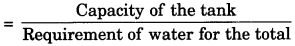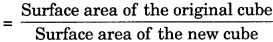# GSEB Solutions Class 9 Maths Chapter 13 Surface Areas and Volumes Ex 13.5

Gujarat Board GSEB Solutions Class 9 Maths Chapter 13 Surface Areas and Volumes Ex 13.5 Textbook Questions and Answers.

## Gujarat Board Textbook Solutions Class 9 Maths Chapter 13 Surface Areas and Volumes Ex 13.5

Question 1.
A matchbox measures 4 cm x 2.5 cm x 1.5 cm. What will be the volume of a packet containing 12 such boxes?
Solution:
Volume of a matchbox = 4 x 2.5 x 1.5 cm3 = 15 cm3
∴ Volume of a packet containing 12 such boxes = 15 x 12 cm3 = 180 cm3

Question 2.
A cuboidal water tank is 6 m long, 5 m wide and 4.5 m deep. How many litres of water can it hold? (1 m3 = 1000 L)
Solution:
Capacity of the tank = 6 x 5 x 4.5 m3 = 135 m3
∴ The volume of water it can hold = 135 m3
= 135 x 1000 L = 135000 LQuestion 3.
A cuboidal vessel is 10 m long and 8 m wide. How high must it be made to hold 380 cubic metres of a liquid?
Solution:
Let the height of the cuboidal vessel be h m.
l = 10 m
b = 8 m
Capacity of the cuboidal vessel = 380 m3
lbh = 380
⇒ (10) (8) = 380 ⇒ h = $$\frac {380}{(10) (8)}$$4.75 m
⇒ h = $$\frac {19}{4}$$ ⇒ h = 4.75 m
Hence, the cuboidal vessel must be made 4.75 m high.

Question 4.
Find the cost of digging a cuboidal pit 8 m long, 6 m broad and 3 m deep at the rate of ? 30 per m3?
Solution:
l = 8 m, b = 6 m and h = 3 m
∴ Volume of the cuboidal pit = Ibh = 8 x 6 x 3 m3 = 144 m3
∴ Cost of digging the cuboidal pit @ ₹ 30 per m3 = ₹ (144 x 30) = ₹ 4320Question 5.
The capacity of a cuboidal tank is 50000 litres of water. Find the breadth of the tank, if its length and depth are respectively 2.5 m and 10 m.
Solution:
Let the breadth of the cuboidal tank be b m.
l = 2.5 m
h = 10 m
Capacity of the cuboidal tank = 50000 litres
= $$\frac {50000}{1000}$$ m3 = 50 m3
lbh = 50
⇒ 2.5 x b x 10 = 50
⇒ 25b = 50
⇒ b = $$\frac {50}{25}$$ = 2m
Hence, the breadth of the cuboidal tank is 2 m.Question 6.
A village, having a population of 4000, requires 150 litres of water per head per day. It has a tank measuring 20 m x 15 m x 6 m. For how many days will the water of this tank last?
Solution:
Requirement of water per head per day = 150 lires
∴ Requirement of water for the total population of the village per day
= 150 x 4000 litres = 600000 litres
= $$\frac {600000}{1000}$$ m3 = 600 m3

For tank:
l = 20 m, b = 15 m and h = 6 m
∴ Capacity of the tank
= 20 x 15 x 6 m3 = 1800 m3
∴ Number of days for which the water of this tank last= $$\frac {1800}{600}$$ = 3
Hence, the water of this tank will last for 3 days.Question 7.
A godown measures 40 m x 25 m x 15 m. Find the maximum number of wooden crates each measuring 1.5 m x 1.25 m x 0.5 m that can be stored in the godown.
Solution:
For godown:
l = 40 m
b = 25 m
h = 15 m
∴ Capacity of the godown = lbh
= 40 x 25 x 15 m3 = 15000 m3
For a wooden crate:
l = 1.5 m
b = 1.25 m
h = 0.5m
∴ Capacity of a wooden crate = lbh
= 1.5 x 1.25 x 0.5 m3 = 0.9375 m3

We have,
$$\frac {15000}{0.9375}$$ = 16000
Hence, the maximum number of wooden crates that can be stored in the godown is 16000.Question 8.
A solid cube of side 12 cm is cut into eight cubes of equal volume. What will be the side of the new cube? Also, find the ratio between their surface areas.
Solution:
Side of the solid cube (a) = 12 cm
∴ Volume of the solid cube = a3
= (12)3 = 12 x 12 x 12 cm3 = 1728 cm3
∴ It is cut into eight cubes of equal volume.
∴ Volume of a new cube
= $$\frac {1728}{8}$$ cm3
= 216 cm3
Let the side of the new cube be x cm.
Then, volume of the new cube = x3 cm3
According to the question,
x3 = 216
⇒ x = (216)1/3
⇒ x = (6 x 6 x 0)1/3
⇒ x = 6 cm
Hence, the side of the new cube will be 6 cm.
Surface area of the original cube
= 6a2 = 6(12)2 cm2
Surface area of the new cube = 6x2 = 6(6)2 cm2
∴ Ratio between their surface areas= $$\frac{6(12)^{2}}{6(6)^{2}}$$ = $$\frac {4}{1}$$ = 4 : 1
Hence, the ratio between their surface areas is 4 : 1.Question 9.
A river 3 m deep and 40 m wide is flowing at the rate of 2 km per hour. How much water will fall into the sea in a minute?
Solution:
In one hour
l = 2 km = 2 x 1000 m = 2000 m
b = 40 m
h = 3 m
∴ Water fall into the sea in one hour = Ibh
= 2000 x 40 x 3 m3
∴ Water fall into the sea in a minute
$$\frac{2000 \times 40 \times 3}{60}$$m3 = 400 m3
Hence, 4000 m3 of water will fall into the sea in tha minute.